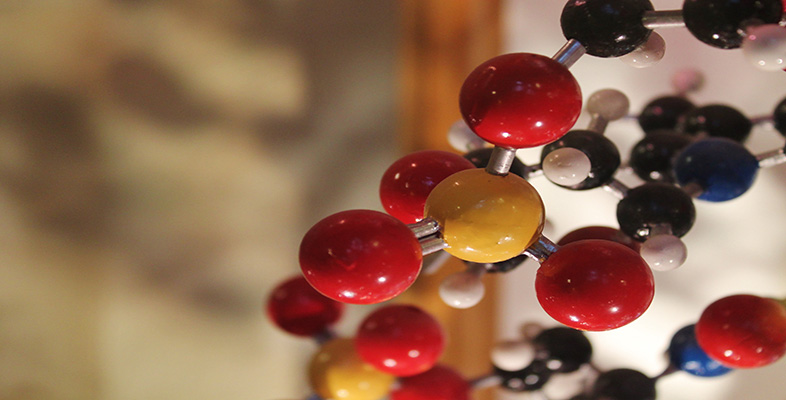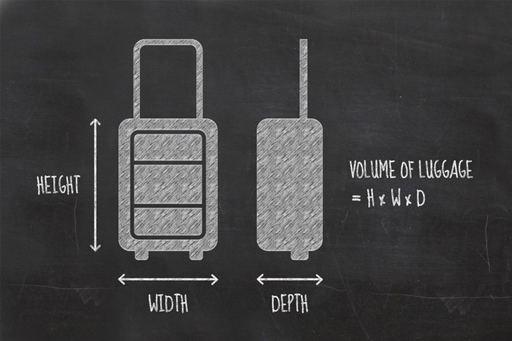Basic science: understanding numbers

Start this free course now. Just create an account and sign in. Enrol and complete the course for a free statement of participation or digital badge if available.

Free course

# 2.3 Calculating volumesFigure _unit2.4.1 Figure 6 Why not work out the volume of your own suitcase?

The volume of something is the amount of space it takes up or contains. A mug can hold a around 0.3 litres of tea, for example. If you take hand luggage onto a flight, you will probably have to check that it fits inside a guide frame.

In the last section, you saw how areas of squares and rectangles are just their two side lengths multiplied together. Similarly, the volume of a cube or cuboid is the lengths of the three sides multiplied together. The units for volume are simply metres multiplied by metres multiplied by metres, or as it is normally written, m3, pronounced ‘metres cubed’.

A rectangular room 3 metres long by 4 metres wide has an area of 12 m2, if the room is 2 metres high, it has a volume of 3 multiplied by 4 multiplied by 2, or 24 m3.

So you can see that there is a pattern:

• Length has one dimension and is measured in m.
• Area has two dimensions and is measured in m2.
• Volume has three dimensions and is measured in m3.